EnchantedLearning.com is a user-supported site.
As a bonus, site members have access to a banner-ad-free version of the site, with print-friendly pages.

 You might also like: Follow-the-Arrows Multiplication Puzzles #5 Printout Follow-the-Arrows Multiplication Puzzles #4 Printout Path-o-Math Multiplication Puzzle #1 Printout Multiplication Printout: 2 Digits x 1 Digit (#1) Follow-the-Arrows Multiplication Puzzles #3 Printout Today's featured page: Leo Connect the Dots Printout

 More Math Activities EnchantedLearning.comMultiplication Printouts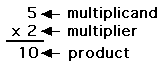The following are multiplication printouts.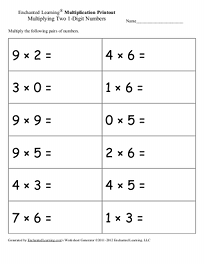Generate Printable Multiplication Worksheets:One Digit x One DigitYou can generate printable multiplication worksheets (one page of questions and one page of answers) with 8, 10 or 12 problems. Every time you click, you get a new worksheet, with a new set of questions and answers. For subscribers only.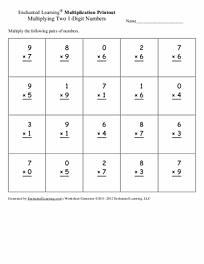Generate Printable Multiplication Worksheets:One Digit x One Digit, Vertical FormatYou can generate printable multiplication worksheets (one page of questions and one page of answers) with 16 or 20 problems. Every time you click, you get a new worksheet, with a new set of questions and answers. For subscribers only.Early Multiplication PrintablesPrintable worksheets and short books that introduce the concept of multiplication to students. The idea of multiplication is developed as the addition of groups of numbers.Early MultiplicationAdding Groups of NumbersAdd groups of items to develop the idea of multiplication as the addition of groups of numbers. Printout #1. Or go to the answers. Printout #2. Or go to the answers. Printout #3. Or go to the answers. Printout #4. Or go to the answers. Printout #5. Or go to the answers. Printout #6. Or go to the answers. Printout #7. Or go to the answers. Printout #8. Or go to the answers. Printout #9. Or go to the answers.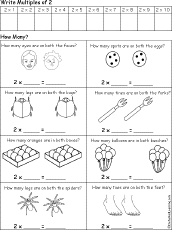Early Multiplication: Count by 2s, 3s, 4s... 9sCount by twos, threes, fours, ... and then write how many objects are in that many groups groups, for example, "How many legs are on all the bugs?" Count by 2s. Or go to the answers. Count by 3s. Or go to the answers. Count by 4s. Or go to the answers. Count by 5s. Or go to the answers. Count by 6s. Or go to the answers. Count by 7s. Or go to the answers. Count by 8s. Or go to the answers. Count by 9s. Or go to the answers.Early MultiplicationDraw Groups of Shapes #1Draw groups of shapes and write equations that describe them. For example, "Draw 2 groups of 3 circles. 2 x 2 = 4" Printout #1. Or go to the answers. Printout #2. Or go to the answers. Printout #3. Or go to the answers.Multiplication: Match Groups of NumbersMatch groups of numbers, for example, match "2 groups of 3"to "2 x 3" to "2 threes." Or go to the answers.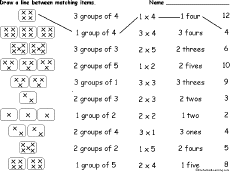Multiplication: Match Groups of Numbers #2Match groups of numbers, for example, match "2 groups of 3"to "2 x 3" to "2 threes." Or go to the answers. Multiplication Table 1-12Print a multiplication table of the numbers 1 to 12.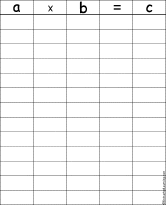Multiplication ChartWrite many equations in which axb=c, like 3x2=6. 1 Digit times 1 DigitMultiply two one-digit numbers. 2 Digits times 1 DigitMultiply two-digit numbers times one-digit numbers. 2 Digits times 2 DigitsMultiply two two-digit numbers.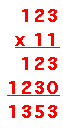Multiplying Integers:3 Digits times 2 DigitsMultiply a three-digit number by a two-digit number.Multiplying Numbers Path-o-Math Puzzles: 2 by 3Make a path through each number matrix so that the product of the numbers is equal to the given answer. Use only horizontal and vertical trails. You may not have to use all the numbers, and don't use a number more than once. Puzzle #1, or the answers Puzzle #2, or the answers Puzzle #3, or the answers Puzzle #4, or the answers Puzzle #5, or the answers Multiplying Decimals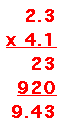Each worksheet has decimal numbers to multiply.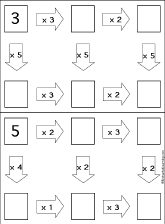Multiplication Follow-the-Arrows Puzzles Do multiplication problems along the paths according to the arrows until you get to the end. You should get to the same final answer three different ways. Puzzle #1, or the answers Puzzle #2, or the answers Puzzle #3, or the answers Puzzle #4, or the answers Puzzle #5, or the answersMultiplication of Fractions Follow-the-Arrows Puzzles Do multiplication of fractions along the paths according to the arrows until you get to the end. You should get to the same final answer three different ways. Puzzle #1, or the answers Puzzle #2, or the answers Puzzle #3, or the answers Puzzle #4, or the answers Puzzle #5, or the answers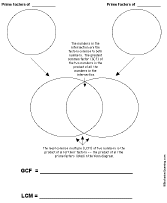LCM, GCFFactor two numbers into primes, then use a Venn diagram to compute their least common multiple (LCM) and greatest common factor (GCF). Multiplying Fractions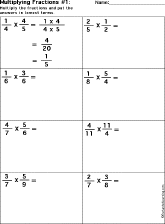Multiply the fractions.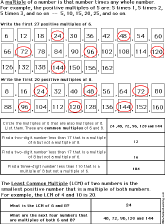Multiples and LCM:Printable WorksheetsWrite multiples of numbers, then determine their least common multiple (LCM). Worksheet #1, or the answers Worksheet #2, or the answers Worksheet #3, or the answers Worksheet #4, or the answers Worksheet #5, or the answers

 +, - EnchantedLearning.comMath x, ÷
 A B C D E F G H I J K L M N O P Q R S T U V W X Y Z
 Number Line Fractions Decimals Measurement Rounding Graphs

Enchanted Learning®
Over 35,000 Web Pages
Sample Pages for Prospective Subscribers, or click below

 Overview of Site What's New Enchanted Learning Home Monthly Activity Calendar Books to Print Site Index K-3 Crafts K-3 Themes Little ExplorersPicture dictionary PreK/K Activities Rebus Rhymes Stories Writing Cloze Activities Essay Topics Newspaper Writing Activities Parts of Speech Fiction The Test of Time iPhone app TapQuiz Maps - free iPhone Geography Game Biology Animal Printouts Biology Label Printouts Biomes Birds Butterflies Dinosaurs Food Chain Human Anatomy Mammals Plants Rainforests Sharks Whales Physical Sciences: K-12 Astronomy The Earth Geology Hurricanes Landforms Oceans Tsunami Volcano Languages Dutch French German Italian Japanese (Romaji) Portuguese Spanish Swedish Geography/History Explorers Flags Geography Inventors US History Other Topics Art and Artists Calendars College Finder Crafts Graphic Organizers Label Me! Printouts Math Music Word Wheels

## Enchanted Learning Search

 Search the Enchanted Learning website for: